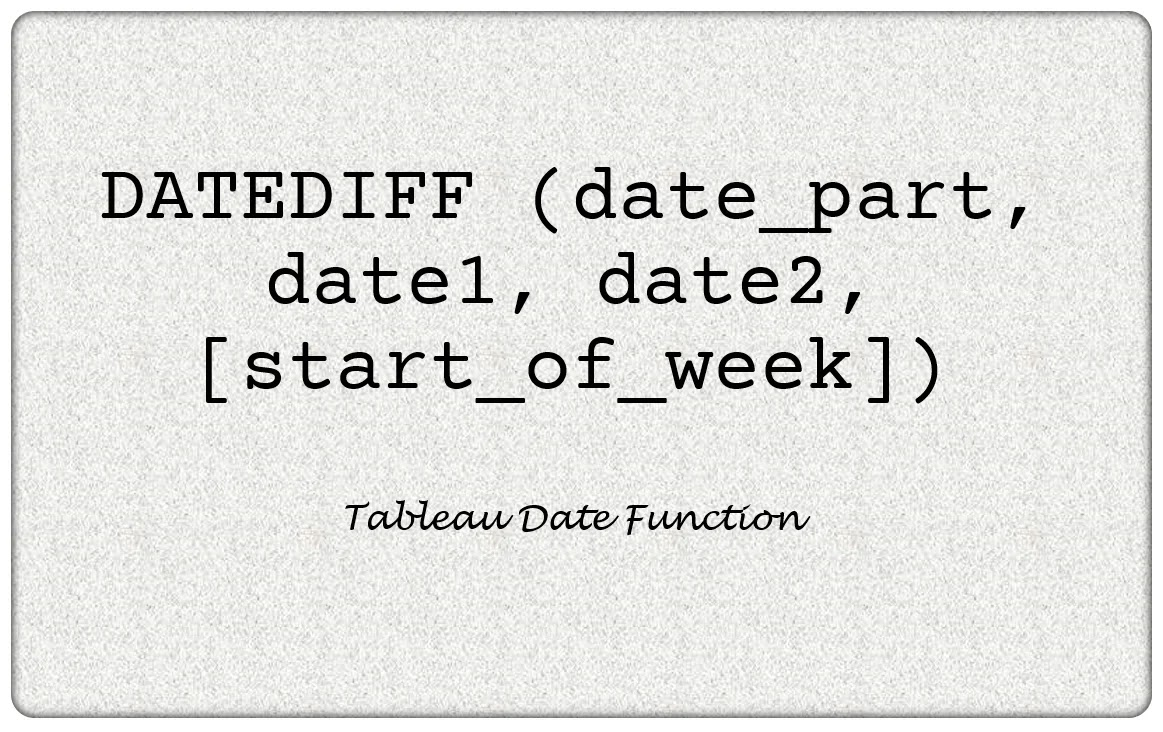# Tableau DATEDIFF Function

×

## Definition

DATEDIFF is a Tableau Date function. It returns the difference between the values of date1 and date2 which are expressed in units of date_part. The start_of_week parameter, which we can use to specify which day is to be considered the first day or the week, is optional. Possible values are 'monday', 'tuesday', etc.
☕ Buy me a coffee! 🙏 Glad my article helped you!×

## How to use DATEDIFF function

The DATEDIFF function returns the difference between the values of two dates expressed in units of date_part. If the [start_of_week] is omitted, it is determined by the data source. DATEDIFF ( date_part, date1, date2, [start_of_week] )
×

## Using DATEDIFF with Unique date_part

The DATEDIFF function returns the difference between the values of two dates expressed in units of date_part. The possible values of date_part from Tableau is listed below.

×

## Calculate days difference with DATEDIFF

The DATEDIFF function below finds the number of days difference between the two dates. DATEDIFF ( 'day', #2020-12-22#, TODAY() )
×

## Calculate weeks difference with DATEDIFF

The DATEDIFF function below finds the number of weeks difference between the two dates. Since both the below dates are in the same week which starts on Monday, it returns 0. DATEDIFF ( 'week', #2020-12-22#, #2020-12-24#, 'monday' )
×

## Calculate YTD with DATEDIFF

The DATEDIFF function can be used to calculate the Year to Date (YTD) value as below. We evaluate an Order Date if the year is same (difference 0) and months is less than or equal to current month to derive the YTD value. IF DATEDIFF ('year',[Order Date],TODAY())=0 AND MONTH([Order Date]) <= MONTH ( TODAY()) THEN [Sales] END

×

## Calculate Previous YTD with DATEDIFF

The DATEDIFF function below finds the number of weeks difference between the two dates. Since both are in the same week which starts on Monday, it returns 0. IF DATEDIFF ('year', [Order Date], TODAY()) = 1 AND MONTH([Order Date]) <= MONTH (TODAY()) THEN [Sales] END

×

## Calculate weeks remaining in current year with DATEDIFF

The DATEDIFF function here calculates the number of weks remaining in current year from a date provided. It is recommend to make all date values below as parameters or dynamic to avoid a yearly manual code update activity. DATEDIFF ( 'week', [Date], #31/12/2020# )
×